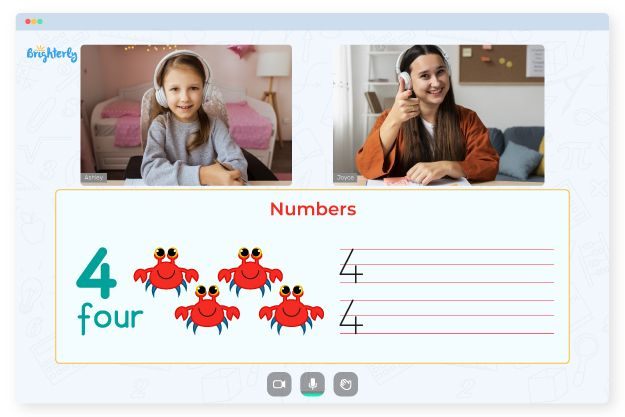# Equations and Inequalities Worksheets

Equations and inequalities are mathematical statements that show the relationship between two expressions. For example, while linear inequality comes from a linear function, an equation is a statement asserting the equivalence of two terms. Some students may be unable to make this distinction, so they need help from resources.

Kids will find problems with inequalities and equations in the inequality equation worksheets. There are also word problems that would require kids to think and understand the tasks before solving them.

## Importance of Solving Equations and Inequalities Worksheet Answers

Understanding equations and inequalities is crucial for grade school students since they will encounter more complex concepts at subsequent levels. Learning the concepts is also essential due to their application in everyday situations.

Inequalities deal with situations with several possible answers, and intervals represent these answers. In solving inequalities, you must have its attributes and principles for solving them in mind. With the equations and inequalities worksheet answers, you can help your kids go confidently from solving simple to more complex inequalities and then on to compound, absolute, and quadratic inequalities.

The worksheet has a stepwise arrangement; kids will start by learning the meaning of inequalities and equations and their application before solving the problems. Solving the expressions equations and inequalities worksheet answers is essential to help students understand and compare numbers and determine the ranges of particular variables.

Students in grades six and above can benefit significantly from solving inequalities questions in free inequalities equations worksheets. Top professionals in mathematics and kids’ learning design these worksheets to ensure that kids learn seamlessly. Furthermore, the resources are colorful and contain images of real-life objects and materials.

Math for Kids

Is Your Child Struggling With Math?
1:1 Online Math Tutoring## Printable Equations and Inequalities Worksheet in PDF

The equations and inequalities worksheets in PDF format are available for download on the Brighterly website. With these materials, you can rest assured that your kids will understand inequalities and equations since the worksheet is play-based.

Also, with the paper format and step-by-step arrangement of questions, your kids will learn independently. Independent and continuous learning will boost kids’ mastery of mathematics.### Equations and Inequalities Worksheet PDF### Equations and Inequalities Worksheet PDF

Equations And Inequalities Worksheet### Equations and Inequalities Worksheet PDF

Inequalities Equations Worksheets### Equations and Inequalities Worksheet PDF

Solving Equations And Inequalities Worksheet Answers

Need help with Numbers?• Does your child need extra support with understanding numbers?
• Start lessons with an online tutor.

Is your child finding it hard to grasp numbers? An online tutor could be of assistance.